# IntroductionSince it's the start of the New Year. I thought of writing a tutorial. For anyone interested to learn Python to start their journey to get a job with it or automate your tasks.

# Getting StartedLet's head on down to Repl.it to create your new repl with Python by selecting `new repl` button and use the dropdown to select `Python`.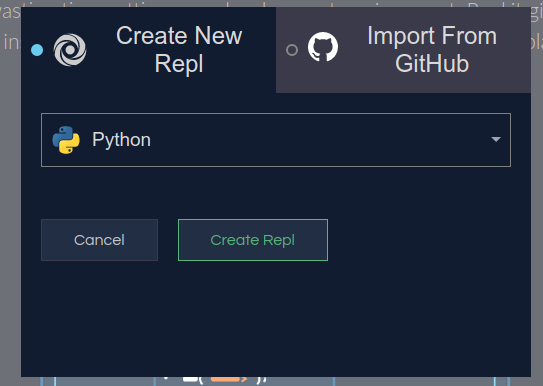Do you have something like this?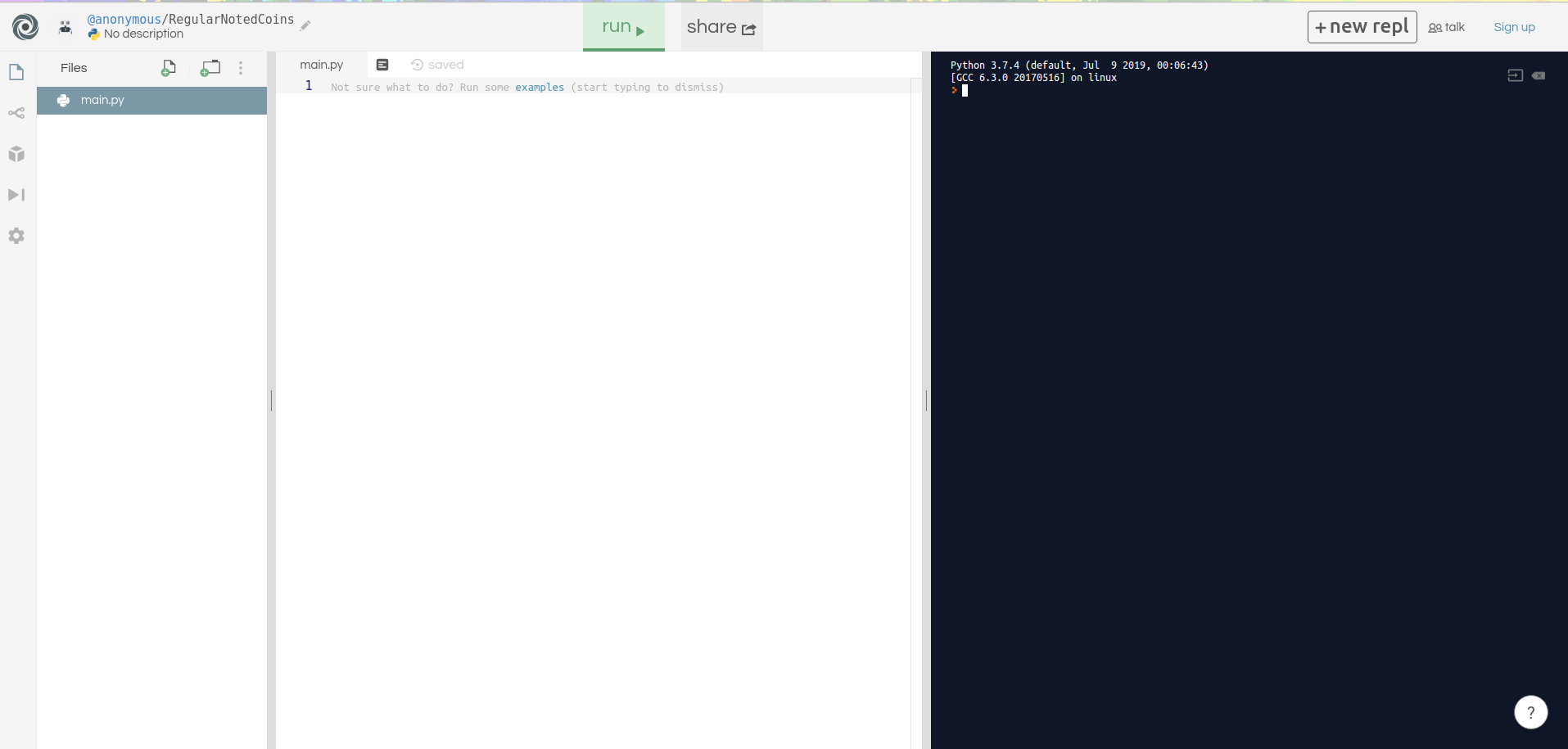Let us enter the codes here in the middle under the file name called `main.py`:

``print('Hello World')``

The `print` statement is used to display words & numbers on your screen. It could be used for debugging purposes as well as you go along in programming in Python.

Great!! Now let us run it by clicking on the `run` button!!! Don't be shy!!! Does it look like this?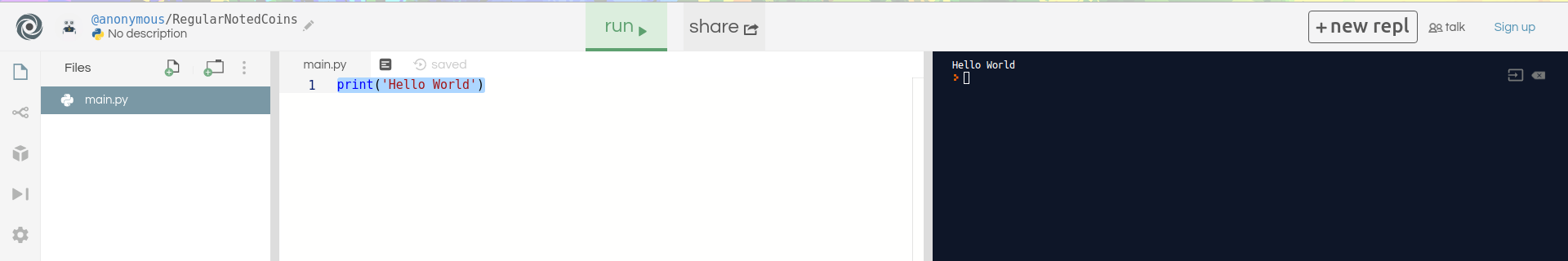Awesome!!! Give yourself a pat on the back for a job well done.

# VariableA variable is a container which you could store something on your computer's memory.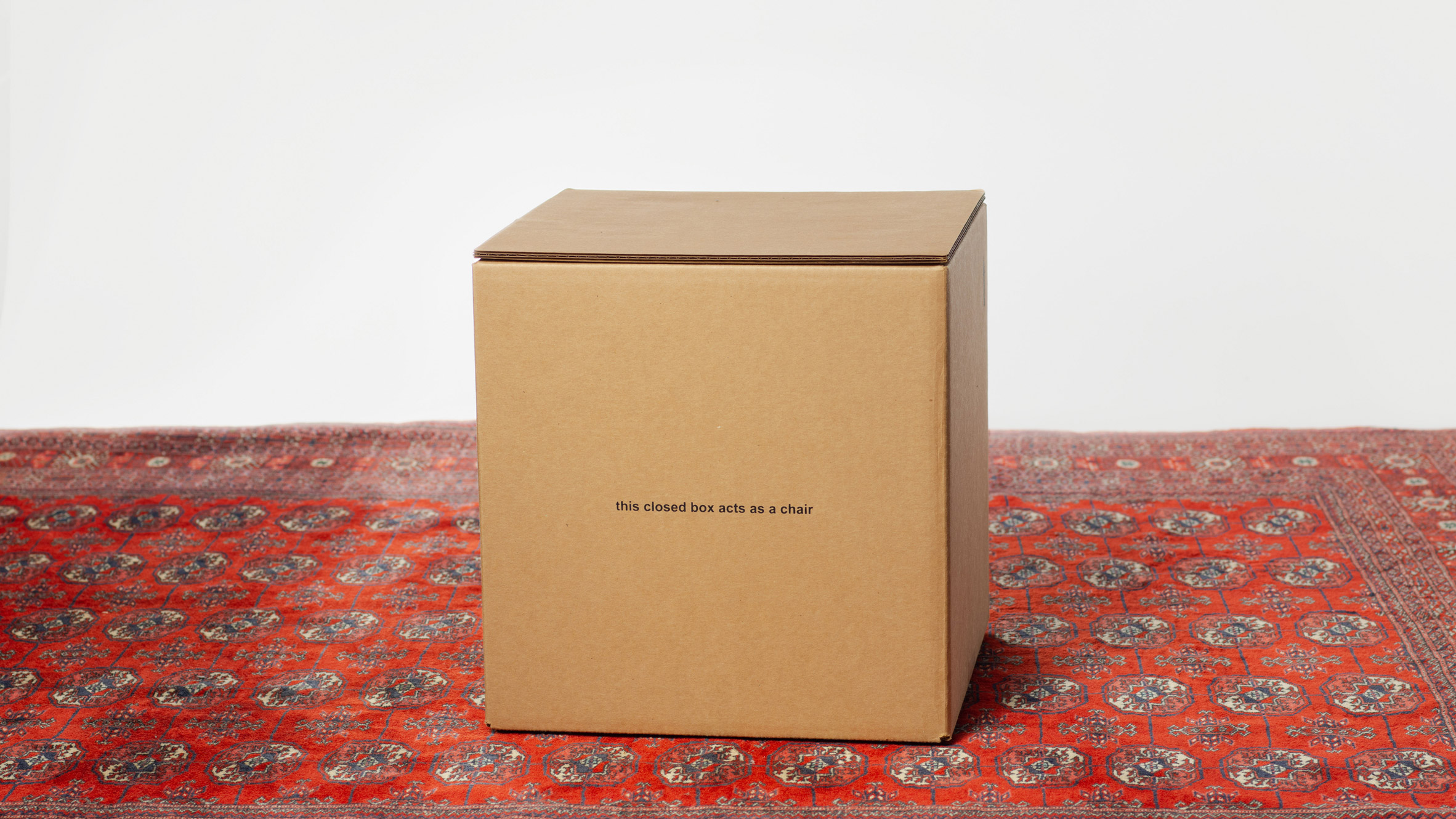Think of it like it is a bottle or a box created by you. It could be used to just store numbers, letters, date and many others.

Here is how it look like to create a variable:

``a = 'Hello World'``

The `a` is the name of the variable, think of it like it's a label for your box.

For the `Hello World`, it is the content that is stored in `a`. Which is called a `string` data type that stores letters, characters or text in it.

``print(a)``

Now try running it on Repl.it, it should look like just two lines of code in Repl.it.

``````a = 'Hello World'
print(a)``````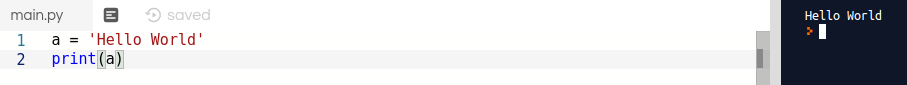## Data Types

Data types are a list of categories on how data is stored in a variable. Here are some common data types, you will be using for Python or other programming languages:

• Integer (int)- whole numbers
``````a = 1
b = 11002
c = -11002``````
• Float (float) - floating-point or decimal numbers
``````a = 1.22
b = 3.221
c = -2918.111``````
• Boolean (bool) - `True` which represents `1` or `False` which represents `0`. This is borrowed from the binary number system.
``````a = True
b = False``````
• Mapping (dict) - It is called a `dictionary` that uses `keys` as a label to get the content. In the example below, `a` is the `key` that retrieves a `value` called "apple".
``````book = {'a': "apple", 'b': 'banna', 'c': 'cat'}
print(book['a'])``````
• Sequence ( tuple or list) - Both `tuple` & `list` are different ways to store data.

It uses an `index` to retrieve a `value` based upon the position of how it is arranged.

It starts with `0` in the first place on the left with an increment of `1` toward the next position.

``````a = (1, 2, 3, 4, 5) # tuple
b = [1, 2, 3, 4, 5] # list

print(a)
print(b)``````

# Functions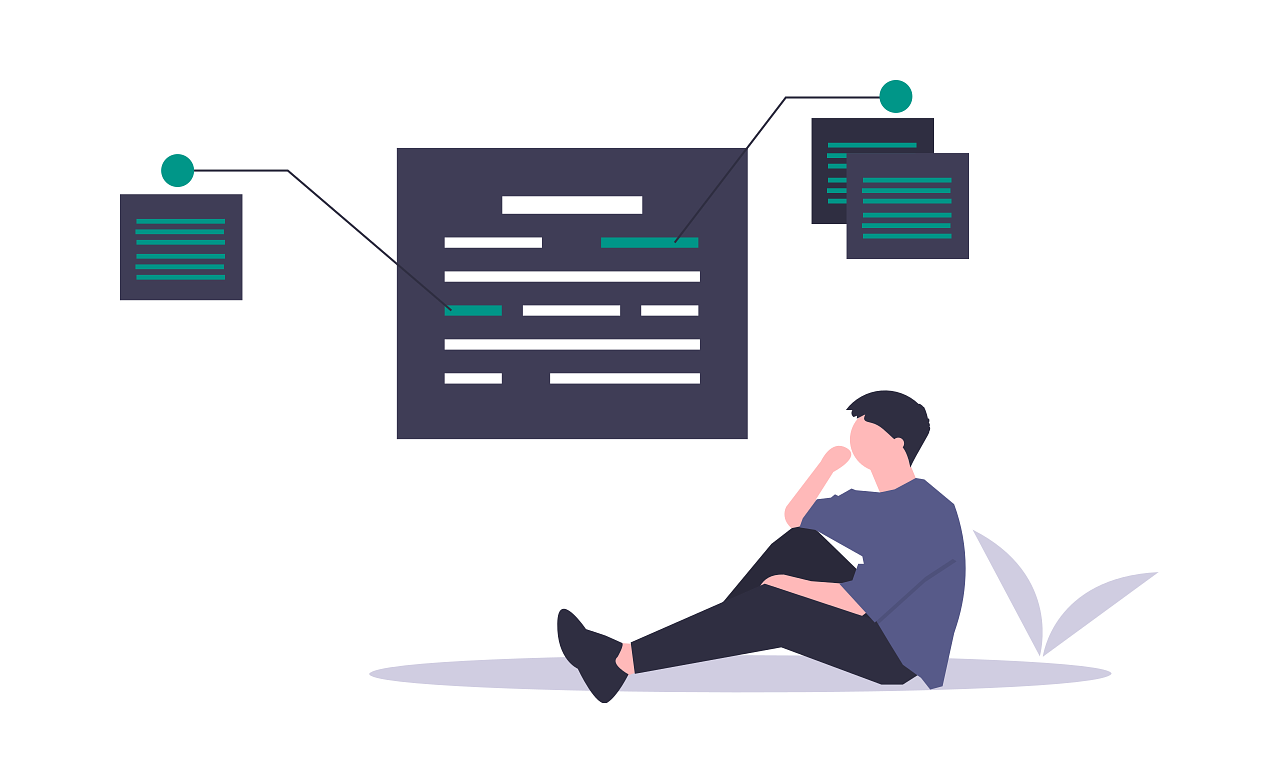Functions are basically pieces of reusable code that are packed nicely in a package. This is how it is declared:

``````def magic():
print('Hello World')``````

You can even add in `variables` as an `input` for your functions to process it. It is called `parameters` or `arguments`:

``````def calculate_area(length, breath):
return length * breath ``````

Now let us call the function, by using the `name` of the function with open and close curly braces like this `()`:

``````def magic():
print('Hello World')

def calculate_area(length, breath):
return length * breath

magic()
print(calculate_area(5,10))``````

Now let us try and run it in Repl.it.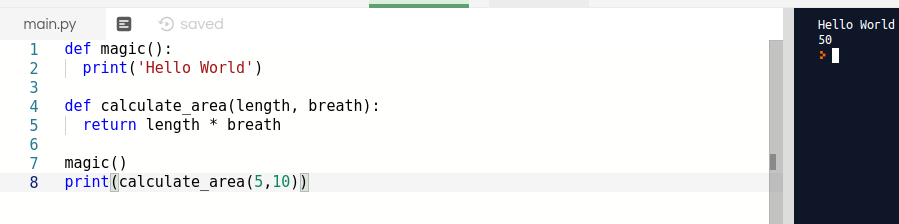# If & Else StatementsThe `If` and `Else` statement is a checklist you had made that starts from the top to the bottom.

## If

The `if` statement checks for certain conditions that meets the expectation and proceeds to execute the piece of code that under the specific condition

You can think of `if` statement like asking a question and you reply if it meets your expectation. Here is an example of an `if` statement:

``````area = 24

if (area == 24):
print('Area is 24')

if (area == 12):
print('Area is 12')``````

The code with `==` means `equals` in Python. Therefore there are two `if` statements.

The first `if` statement checks if `area` is equal to `24`, whereas another is checking `if` the area is `12`.

## Else

For the `else` statement, it is sort of like the backup plan when your `if` statement fails to get the correct condition:

``````area = 24

if (area == 10):
print('The area is 10')
else:
print('The area is not 10')``````

## Else If

An `elif` statement represents something like having `multiple plans` starting from the first to the last.

This is different from the previous two `if` statements. Since there is a flow which they depend on the `if` or `elif` to fail. Before proceeding to the next `elif` or `else` statement:

``````area = 24

if (area == 10):
print('The area is 10')

elif (area == 12):
print('The area is 12')

elif (area == 20):
print('The area is 20')

else:
print('Area is more than 12')``````

This is a way of programming called `if-else-if` ladder that makes decisions in your program.

# ForThe `for` statement or `for loop` is used to repeat a piece of code multiple times.

Here is an example of how it looks like in declaring and creating a `for` loop statement:

``````for i in range(11):
print(i)``````

Now try running this code before proceeding with my explanation. Does it look like this?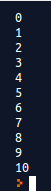Alright, let me explain how this is done. The `i` is a variable. Think of it like it is the head of a crawling machine that grabs a value and keeps it temporary.

Now `range` is a function which returns a sequence of numbers. This starts with `0` (default) and increments by `1` till the last position.

This essentially repeats a code for `11` times which is the number that you had included in the `range` function for the `for` loop.

To customise your for loop, you can make changes to the `range` to provide you with a different result:

``````for i in range(1, 11):
print(i)``````

Now try running again what do u see? Is it something like this 1?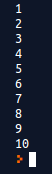Now in the `range`, you had included 2 parameters. The first one `1` as the number for `i` to `start` and `11` as the ending which is why it returns `10` times.

You can control the increment as well instead of just the default `1` for the `range` function you can add a 3rd parameter that represents the increment in the right side.

``````for i in range(1, 11, 2):
print(i)``````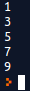# ConclusionPhew.....that was a lot of information to digest, congrats in making it through the end of the tutorial. I hope you had gained useful insights into it.

Lastly, I suggest that you jump over to Clever programmer's 11 hrs tutorial on Python for the absolute beginner. He offers tons of insights to get a job or getting your feet wet as a Python freelancer.